Get instant live expert help with Excel or Google Sheets“My Excelchat expert helped me in less than 20 minutes, saving me what would have been 5 hours of work!”

#### Post your problem and you’ll get expert help in seconds.

Your message must be at least 40 characters
Our professional experts are available now. Your privacy is guaranteed.

# If number is between two values

We are able to check if a number is between two values by using the AND function.  AND evaluates all logical tests and returns TRUE only if all conditions are met.  Otherwise, it returns FALSE.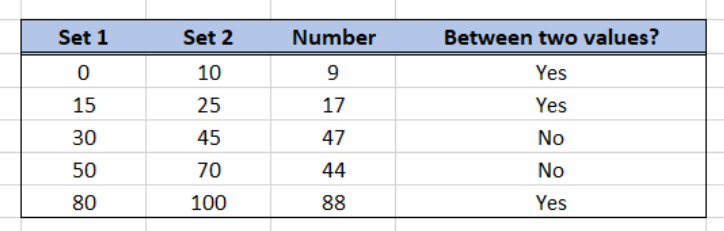Figure 1.  Final result: If number is between two values

## Syntax of AND

`=AND(logical1, [logical2], ...)`

• Only logical1 is required while succeeding logical tests are optional
• logical1, logical2,…etc. are logical tests that the AND function will evaluate to be either TRUE or FALSE

## Check if a number is between two values

In order to check if a number is between two values, we can use the AND function with two logical tests.

• Enter the formula in E4:
`=AND(D4>B4,D4<C4)`

The first logical test D4>B4 evaluates if the number is greater than the value in Set1, while the second logical test D4<C4 evaluates if the number is less than the value in Set2.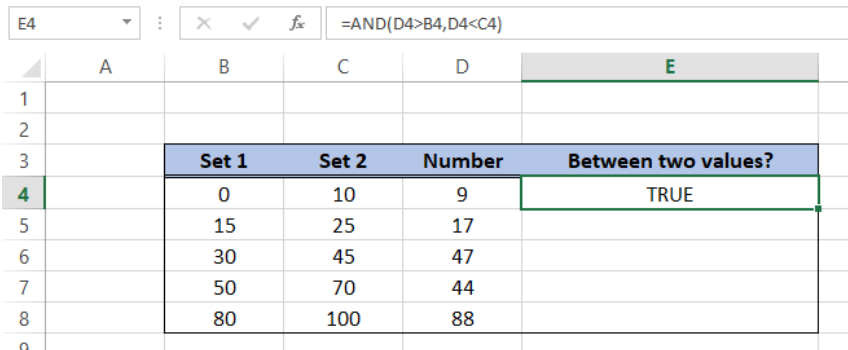Figure 2.  Entering the formula using AND

• Select E4 and drag the formula down to E8

As a result, we have determined that the number in rows 4, 5 and 8 are between the values in Set 1 and Set 2.  For example, the number “9” in D4 is between the values 0 and 10. Hence, the AND function returns TRUE.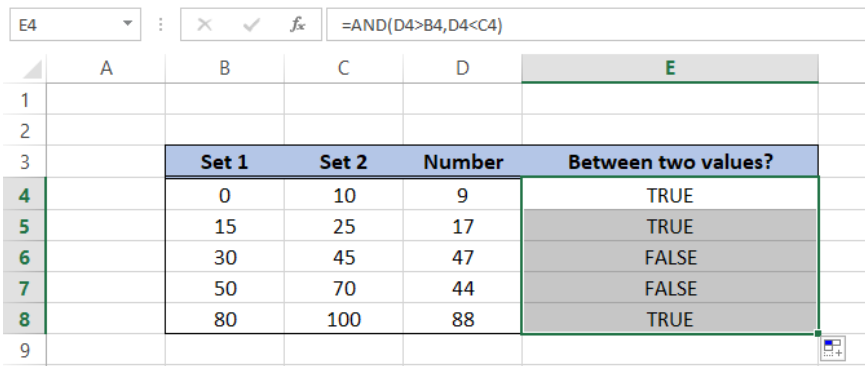Figure 3.  Output: If a number is between two values

However, there are times when the values we are comparing are interchanged and are not arranged in ascending order.  Suppose we have below data wherein Set2 values are not always greater than the values in Set1.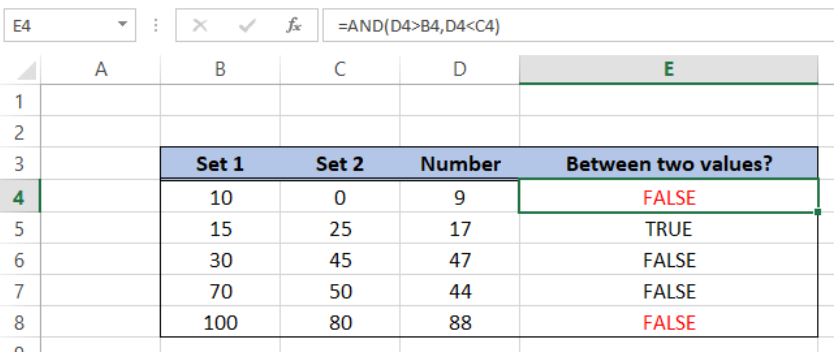Figure 4.  Erroneous results when values are interchanged

Using the same formula will yield erroneous results.  Hence, we need to apply a formula that compares if a number is greater than the smaller value, and evaluates if a number is less than the larger value.  This time we use the AND function with MIN and MAX.

Check if number is between two values using MIN and MAX

• Enter the formula in E4:
`=AND(D4>(MIN(B4,C4)),D4<(MAX(B4,C4)))`
• Select E4 and drag the formula down to E8

Our formula first compares the number with the smaller of the two values through the MIN function.  Next, it compares the number with the larger of the two sets of values through the MAX function.

Below table shows the correct results when evaluating if a value is between two numbers in Set1 and Set2, regardless of which set contains the smaller or larger number.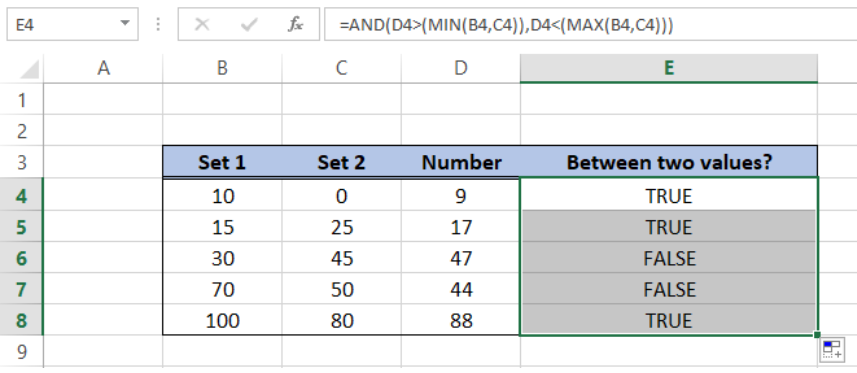Figure 5.  AND formula with MIN and MAX

## IF statement between two numbers

We can customize the result when comparing values by using the IF function with the AND function.  Suppose we want the formula to return “YES” if a number is between two values, and “NO” if otherwise.

• Enter the formula in E4:
`=IF(AND(D4>(MIN(B4,C4)),D4<(MAX(B4,C4))),"Yes","No")`
• Select E4 and drag the formula down to E8

We simply wrapped the IF function around the AND formula.  As a result, the formula returns “Yes” instead of “TRUE”, and “No” instead of “FALSE”.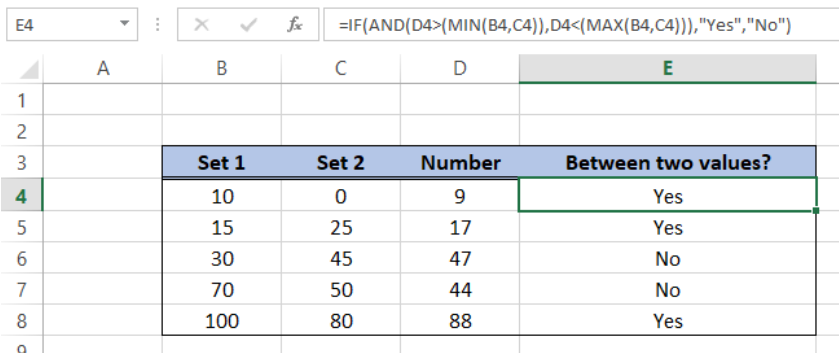Figure 6.  Output: IF statement between two numbers

## Instant Connection to an Excel Expert

Most of the time, the problem you will need to solve will be more complex than a simple application of a formula or function. If you want to save hours of research and frustration, try our live Excelchat service! Our Excel Experts are available 24/7 to answer any Excel question you may have. We guarantee a connection within 30 seconds and a customized solution within 20 minutes.